# How to Multiply Fractions with Easy Steps & 7 Engaging Activities

All PostsYou’ve already succeeded in teaching multiplication, but now comes the seemingly daunting task of teaching them how to multiply fractions.

Deep breaths.

Teachers and students alike might argue this concept is more daunting than leaping from subtraction and addition to multiplication.

Fortunately, strategies exist that should make learning how to multiply fractions much easier to grasp — and we’ve compiled them for you in a clear-cut guide.

## The Simple Way To Multiply Fractions

There are three simple steps your students need to follow when learning how to multiply fractions:

1. Multiply the numerators (top numbers)
2. Multiply the denominators (bottom numbers)
3. If needed, simplify or reduce the fraction

Let’s go through an example together!

### Multiplying fractions example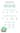## Understanding the 3 types of fractions and how to multiply them

Before we go deeper on this concept and explain multiplication of fractions, we thought it would make sense to understand the different types of fractions.

A good place to start is with some simple terminology around how a fraction is composed:

• Numerator — the top number, which refers to how many parts (of a whole) you have.
• Denominator — the bottom number, which refers to the total number of parts making up the whole.Credit: Brett Berry

Now we’ll go into the three types of fractions and how to multiply them.

### 1. Multiplying proper fractions

A proper fraction has a numerator less than the denominator. This is the easiest place to start when multiplying fractions. The example we used above is perfect for multiplying this type of fraction.

For example: ½, ⅔, ¾, ⅘, ⅚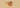### 2. Multiplying improper fractions

Though similar in structure, an improper fraction has a numerator greater than the denominator.

Note: When a numerator is equal to the denominator, it’s considered “improper” because you can change it into a whole number. The same rule applies to improper fractions such as ²⁶⁄₁₃ which, if reduced, become whole (i.e., two).

For example: ³⁄₂, ⁵⁄₃, ⁷⁄₆, ¹¹⁄₁₀, ⁸⁄₈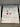Credit: Miranda Weenusk

You multiply improper fractions the same way you do proper ones. However, sometimes students can end up with improper fractions.

Take this problem as an example:

Solve: ⁵⁄₃ × ⁷⁄₆ Multiply numerators: 5 × 7 = 35 Multiply denominators: 3 × 6 = 18 New fraction: ³⁵⁄₁₈

If students are familiar with mixed fractions, they can change the improper fraction to a mixed one. In this case, that mixed number would be 1 ¹⁷⁄₁₈.### 3. Multiplying mixed fractions

Before teaching students how to multiply fractions with mixed numbers, there are three steps they should know:

1. Convert any mixed fractions into improper fractions
2. Multiply the improper fractions
3. Convert the final product back into a mixed number

To complete the first step, teach your students how to make a mixed number “MAD”.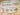Credit: Fabulous Finch Facts

Remember: a mixed number is composed of a whole number and proper fraction. To complete step one and convert a mixed fraction into an improper one, you need to:

• Find the new numerator -- Multiply the whole number with the denominator, then add the original numerator to it.
• Keep the same denominator -- The denominator remains unchanged.

Step two, multiply the improper fractions as we illustrated before this section.

Step three, convert that improper fraction back into a mixed number. Here’s a little rhyme to help your students remember how to do this:

With an improper fraction, division is the action!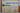Credit: Heather’s Show and Tell

Let’s use the example pictured earlier: ¹⁶⁄₅.

If division is the action, then you need to divide the numerator (16) by the denominator (5) and see if there’s a remainder.

Five goes into 16 three times evenly, for a total of 15. That means the whole number portion of the mixed number will be three.

But it didn’t divide perfectly, which you can see by the remainder of one. So, the fraction portion of the mixed number is the remainder over the denominator of the original fraction (⅕).

What that looks like as a mixed fraction is 3 ⅕.

For a more visual walkthrough of how to multiply mixed numbers, watch this four-minute video from Khan Academy:

And that’s what you need to know when multiplying fractions and mixed numbers.

## What about multiplying fractions with whole numbers?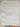Credit: Ericka Najera

Multiplying whole numbers and fractions may stump your students. Why? Because it seems like there’s only one fraction instead of two.

But when learning how to multiply fractions by whole numbers, students can rewrite them as fractions. Instead of the 4, for example, turn it into the fraction ⁴⁄₁.

Now it’s easier and clearer to multiply. For example, they can rewrite 2 × ⁵⁄₁₃:

Solve: 2 × ⁵⁄₁₃ Rewrite whole number as a fraction: ²⁄₁ × ⁵⁄₁₃ Multiply numerators: 2 × 5 = 10 Multiply denominators: 1 × 13 = 13 New fraction: ¹⁰⁄₁₃

Note: If students struggle with whole numbers, explain that they can think of a whole number as a top number, with the bottom number always being one.

## Area models for fraction multiplication

Perfect for the visual learners in your class, the area model effectively illustrates what one fraction times (or “of”) another looks like.

As you can see from the illustration below, creating an area model when multiplying fraction is easy:

1. Draw the fractions you’re multiplying in separate boxes, each using a different color
2. Combine the drawings into one box, using a new color for the parts that overlap
3. To write the product, ask yourself two questions:
How many boxes have both colors? This will be your numerator
How many boxes are there in total? This will be your denominator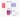Credit: Mrs. Coggins

### A catchy reminder

Oh! And if your students ever forget the steps, just remind them to sing this song:

Multiplying fractions? That’s no big problem. Do top times top over bottom times bottom. And before you say goodbye, don’t forget to simplify!

## 2 Major errors students make with fraction multiplication

Though some students will quickly grasp your fraction multiplication lessons, others can struggle with these new concepts.

The earlier teachers catch these misconceptions, the sooner students can learn from and correct their errors.

According to the What Works Clearinghouse Institute of Education Sciences practice guide, “Developing Effective Fractions Instruction for Kindergarten Through 8th Grade,” these are some of the most common misconceptions in regards to learning how to multiply fractions.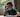### 1. Believing whole numbers have the same denominator as fraction in a problem

The guide’s panel of eight experts recognized this misconception can lead students to take a problem such as 4 – ⅜ and rewrite it as ⁴⁄₈ – ⅜, for an incorrect answer of ⅛.

When presented with a mixed number, students with such a misconception might add the whole number to the numerator, as in ³¹⁄₃ × ⁶⁄₇ = (³⁄₃ + ⅓) × ⁶⁄₇ = ⁴⁄₃ × ⁶⁄₇ = ²⁴⁄₂₁.

Helping students understand the relation between mixed numbers and improper fractions -- and how to translate each into the other -- is crucial for working with fractions.

Avoid the temptation to blast through foundational lessons.

Take the time your students need to help them understand the relationship between improper fractions and mixed numbers, and how to convert them from one to the other.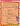Credit: Once Upon a Creative Classroom

### 2. Leaving the denominator unchanged

Students can make the mistake of forgetting to multiply equal denominators. This is likely due to the fact you don’t have to touch equal denominators in fraction addition.

For example, they might see ⅔ × ⅓ and incorrectly answer ⅔ instead of ²⁄₉.

In the practice guide, expert panelists suggest “explaining the conceptual basis of fraction multiplication using unit fractions (e.g., ½ × ½ = half of a half = ¼).”

In particular, teachers can show that the problem ½ × ½ is actually asking what ½ of ½ is, which implies that the product must be smaller than either fraction being multiplied.

Verbalizing this misconception is helpful, but visualizing it is especially effective. Enter the fraction wall!

Fraction walls are a brilliant way to help students see what, in this case, an abstract one half of one half (i.e., one quarter) looks like.

Now you’re aware of many students’ pain points while learning how to multiply fractions, what’s next? Let’s explore ways to make your fraction lessons stick — and why worksheets may not be the best strategy.

### Do worksheets help with multiplying fractions?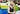Dr. Stephen Camarata, a specialist in child development and developmental disabilities, believes children’s natural curiosity is being derailed.

In an essay for Psychology Today, he expressed discontentment with the presumption that completing worksheets is directly associated with improvements in learning.

But the data on US achievement scores as compared to the rest of the world indicate otherwise. Ironically, as more and more worksheets are pushed in earlier and earlier grades and the more rote, boring homework is forced on developing minds, student outcomes in the US decline further.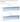Source: Source: NAEP Data Explorer, National Center for Education Statistics

A Pew Center for Research report indicated a decline in already dismal US achievement scores. Dr. Camarata highlighted:

Only 34% of fourth graders and 27% of eighth graders were rated as proficient in math in 2011 and this declined to 33% for 4th graders and 25% for 8th graders in 2015 (the most recent year these data are available).There is no way to put a positive spin on these outcomes: Currently, more than two-thirds of fourth-graders and three in four eighth graders are not proficient in math. This ranks 38th in the world.

Does this mean schools should go worksheet-free? Not necessarily.

Correlation isn’t causation. In fact, many teachers and students have found success with worksheets.

However, educators need to realize education is rapidly changing, from worksheets to classroom technology.

So, here are a few creative ways to teach fraction multiplication — worksheet-free!

## 7 Engaging activities to teach students how to multiply fractions

### 1. Prodigy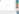Prodigy is a curriculum-aligned online math game that offers content from every major math topic from 1st to 8th grade.

Along with many other skills and standards, Prodigy can help students learn how to:

• Multiply two fractions
• Multiply a fraction by a whole number
• Multiply two fractions via word problems
• Multiply a whole number by a missing fraction

And more!

Playing Prodigy can take your students on a fantasy-inspired learning journey that’s both fun and educational. Plus, you'll get data on their progress to use in your everyday teaching strategies.

### 2. Flip-it fractions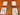Credit: MathFileFolderGames

For this activity, split your students into groups of four. Next, they’ll divide themselves in teams of two, one being Player A and the other Player B.

Give each group a deck of shuffled cards (aces = 1, jacks = 10, queens = 11, and kings = 12).

As in the picture above, each student will draw a numerator card (above the pencil) and a denominator card (below the pencil).

Both Player A’s will rewrite and multiply the fractions on paper, then simplify the product if possible. Once they’ve answered, Player B’s will do the exact same thing.

Depending on your schedule, you can assign the whole deck or give students a timer to complete as many as they can.

Have students hand in their answer sheets after the activity for you to mark, or go through 10+ questions together as a class.

Note: You can also call this game “Slam-it fractions,” replacing the cards and pencils with dominoes.

### 3. Fraction multiplication BINGO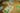Credit: jimmiehomeschoolmom

Every student gets a fraction-filled bingo card along with small sheets of paper (or “bingo chips”) on which are corresponding fraction multiplication problems.

When you say “GO,” they can start solving each problem one by one, laying the chip on top of the correct fraction.

It’s up to you whether you want them to complete a line or the entire bingo card. You can also choose whether or not the bingo card answers are simplified.

For simplicity’s sake, you can give everyone the same bingo card with the same questions. That way, you can go through each problem afterwards and walk through how to solve them together.

Note: You can use this example and the ones that follow as formative assessments to gauge student learning and catch misconceptions early

### 4. Word problems

Word problems are a wonderful way to make math lessons relevant to your students’ lives.

Learning how to multiply fractions may seem foreign to them, but a simple story can change their entire perspective not just about fractions, but math as a whole.

Here’s a word problem example:

You have ½ a bag of chips in the cupboard, but ate ½ of it after dinner. How much of the whole bag did you eat? (Do not reduce your answer to the lowest terms.)

Granted, it’s a simple example. But a second ago, that fraction was just a number above and below a short line. Now, however, this “everyday” word problem has made multiplying fractions applicable to real life.

### 5. Fraction war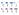Credit: Sherry Fisher

This two-person activity is adapted from the card game “War.”

Note: Many teachers are moving away from speed-based problem solving because it doesn’t necessarily demonstrate student understanding. You might also have kids in your class who get anxious under the pressure of doing math quickly and thus underperform. So, it’s best to gauge your class before playing this version.

Sitting side by side, each student will have half a deck of fraction cards (which you can download here).

With their pile of cards facedown, each student will draw a card at the same time.

The first student who multiplies the two fractions correctly adds those cards to their deck.

A student wins if they end up with the entire deck of fraction cards in their hand, or they have the majority of cards at the end of, for example, 20 minutes.

What’s more, the quick think-on-the-spot nature of this fraction activity can help improve your students’ mental math skills.

Alternatively, you can run a version of this game allowing everyone to play against you.

Who doesn’t love a chance to outsmart their teacher!?

Split your class into five groups.

You’ll go to the first group and have a one-question face-off, before moving to the second group and so on. Remember: the point of this version isn’t to answer before your students, but to help boost their mental math abilities.

This allows students to answer collectively, so as to avoid singling kids out who may be struggling with mental math.

### 6. Food fractions

Kids love food -- it’s no secret! So why not incorporate it into your lesson plan?

A teacher in the Tweet above got her kids to practice how to multiply fractions by converting delicious food recipes.

You can have each student choose their favorite food and multiply the ingredients to feed the entire class.

An incentive might help, too! For example, once everyone has converted their favorite food, choose a safe snack the class will love.

Pull up the original recipe. Now have your students work together to multiply ingredients and, if they do it properly, the whole class will get a homemade (or store-bought) baked good!

### 7. Multiplied fraction pennant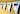Credit: Scaffolded Math

Cut out ribbon- or triangle-shaped sheets of paper for each student. At the top, write “I can multiply…”

Below that, you’ll include:

• An area model illustrating the fractions they’re multiplying
• The multiplication problem itself (with space to show their work)
• A space at the bottom that reads “My product reduces to...”

Once every student has completed and decorated their fraction multiplication pennant, glue or tape them to a string!

Not only will this active learning activity help enforce how to multiply fractions, but it’ll give your students a confidence boost. It’s their work up on the wall, a problem they solved, and everyone can see it.

## Excited to teach your students how to multiply fractions now?

We hope so!

Multiplying fractions can be a daunting task — to learn and teach.

Hopefully the thorough breakdown of different types of fractions, how to multiply them, and how to make teaching them fun helps enrich you and your students’ teaching and learning experience.